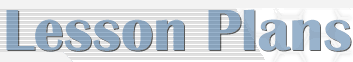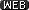Math Portal Home > Middle School Lesson Plans

 8th Grade Lesson Plans - The activities included in this section are models for lesson plans that exemplify best practice teaching in mathematics. Sources for the activities include Pinellas County Secondary Mathematics teachers, the Jump Start Algebra Summer Program, and the Opening the Gate project, a statewide funded initiative that began in 1992. Teacher and student versions of each activity are provided. These lesson plans can be viewed through the web links below shown by thebuttons.Baseball Proportion: Teacher Notes - proportional reasoning, scale factor, similarity, solve proportionsBaseball Proportion: Student WorksheetBungee m&ms: Teacher Notes - data collection, graphing, line of best fit, interpret data, organize data, make predictionsBungee m&ms: Student WorksheetCylinder: Teacher Notes - Diameter, Radius, Area, Volume, Ratio, A = pr2, V = pr2hCylinder: Student WorksheetDrops on a Penny: Teacher Notes - data collection, graphing, line of best fit, interpret data, organize data, make predictionsDrops on a Penny: Student WorksheetFamous People: Teacher Notes- estimation, graphingFamous People: Student WorksheetFamous People: Birth DatesGet a Half Life: Teacher Notes - data collection, graphing calculator, line of best fit, interpret data, functions, calculator commands, exponential decay, graph data, curve of best fitGet a Half Life: Student WorksheetGo Fish: Teacher Notes - data collection, interpret data, proportional reasoning, solve proportionsGo Fish: Student WorksheetGraphing Calculators: Teacher NotesGraphing Calculators: Student WorksheetHuman Coordinate Plane: Teacher Notes- coordinate plane, axis, slope, intercept, graphing coordinate pairs, representing lines and inequalitiesHuman Coordinate Plane: Student WorksheetA Human Number Line: Teacher Notes- number line, symbols, equality, inequality, addition, subtraction, solving equations and inequalitiesA Human Number Line: Student WorksheetLine Up: Teacher Notes - integers, radicals, scientific notation, relationship of numbers, simplify and order numbers and expressionsLine Up: Student WorksheetReflection: Teacher Notes - transformations, reflections, coordinate plane, graphing points, making transformations (flips, sides, turns)Reflection: Student WorksheetThe Shadow Knows: Teacher Notes - ratios, proportions, measurement, measuring lengths, solving proportionsThe Shadow Knows: Student WorksheetShaquille O'Neal Hand/Foot Span: Teacher Notes - data collection, interpret data, line of best fit, organize data, graph data, make predictions, ratiosShaquille O'Neal Hand/Foot Span: Student WorksheetSpaghetti Bridges: Teacher Notes - data collection, graphing, linear equations, interpret data, organize data, graph data, make predictionsSpaghetti Bridges: Student WorksheetTwo for One Box Company: Teacher Notes - volume, nets, ratio, V=lwhTwo for One Box Company: Student WorksheetViewing Tubes: Teacher Notes- data collection, graphing, line of best fit, interpret data, organize data, graph data, make predictionsViewing Tubes: Student WorksheetWacky Water World: Teacher Notes - linear equations, graphing calculators, interpret data, y=mx+b, graphing linear equations, graphing calculator commands, make predictionsWacky Water World: Student WorksheetWalking the Plank: Teacher Notes- data collection, graphing calculators, interpret data, y=Mx+b, graphing linear equations,, make predictionsWalking the Plank: Student Worksheet back to top
 Math Portal Home > Middle School Lesson Plans